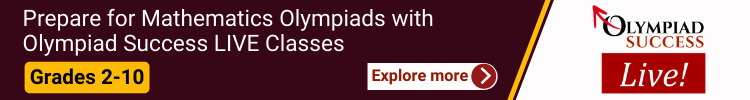All fields are required

We help students of class 1 to 10 in preparation of IMO exam and courses through sample question and practice papers.

International Maths Olympiad (IMO) helps students grow their analytical thinking. IMO develops the ability to think faster & effectively to find the solutions by thinking of a whole coherent process.

IMO Syllabus & Marking Scheme

Class

No. of Questions

Marks

1-4

35

50

5-10

50

50Schedule for 2019-2020 examination

The exam will be held on 28th November 2019 and 19th December 2019.

Benefits

• Indian Talent Olympiad is a highly competitive exam which helps students gain knowledge & prepares them for the competitive world ahead.
• Our International-level examination is designed in close co-ordination with experienced teachers, trainers and educationists which aims to develop strong conceptual foundation of subjects, sharpen analytical thinking and problem solving skills. Over the last years, it has striven to spread a proper understanding of all the subjects like Science, Mathematics, English, General Knowledge, Computer, Art (Drawing), Essay and Social to young generation of the country.
• Awards & Prizes worth Rs. 5 Crore.*

##### Class 1

Section - 1 : Shapes And Space, Number System, Addition, Subtraction, Money, Time, Measurement, Logical Reasoning.

Section - 2 : High Order Thinking Question - Syllabus as per Section 1.

Section - 3 : Patterns, Grouping of Figures, Ranking Test, Measuring Units, Geometric Shapes, Odd one out, Analogy, Spaal Understanding, Symmetry.

##### Class 2

Section - 1 : Lines and Shapes, Number system, Addition, Subtraction, Time, Money, Measurement, Logical reasoning, Model Question Paper.

Section - 2 : High Order Thinking Question - Syllabus as per Section 1.

Section - 3 : Patterns, Grouping of Figures, Ranking Test, Measuring Units, Geometric Shapes, Odd one out, Analogy, Coding Decoding, Symmetry, Embedded Figures.

##### Class 3

Section - 1 : Shapes & figure, Number system, Addition & Subtraction, Multiplication & division, Money, Measurement, Time, Calendar, Fraction, Logical reasoning, Model Question Paper.

Section - 2 : High Order Thinking Question - Syllabus as per Section 1.

Section - 3 : Alphabet test, Number series, Analogy and Classification, Ranking test, Logical sequence of words and leers, Coding decoding, Blood Relations, Direcon sense test, Mirror Image, Geometrical shapes, Grouping, Embedded, Days and dates and possible.

##### Class 4

Section - 1 : Shapes and Sizes, Number system, Addition, Subtraction, Time, Money, Measurement, Logical reasoning, Model Question Paper.

Section - 2 : High Order Thinking Question - Syllabus as per Section 1.

Section - 3 : Alphabet, Number series, Analogy and Classification, Ranking, Logical sequence, Coding decoding, Paper Folding and Cutting, Blood Relations, Direcon sense, Mirror Image, Geometrical shapes, Grouping, Embedded, Days and dates and possible.

##### Class 5

Section - 1 : Concept of Geometry, Number System, Factors & Mulples, Addition & Subtraction, Multiplication & Division, Fractions & Decimals, Measurement, Data Handling, Logical reasoning, Model Question Paper.

Section - 2 : High Order Thinking Question - Syllabus as per Section 1.

Section - 3 : Analogy, Mirror and Water Images, Direcon Sense, Ranking, Puzzle, Coding-Decoding, Venn Diagram, Series, Blood Relations, Figure Matrix, Mathemacal Operations, Alphabet test, Cubes and Dice.

##### Class 6

Section - 1 : Concept of Geometry, Number System, Factors & Mulples, Integers, Decimal Fractions, Rao & Proporon, Arithmec, Basic Algebra, Data Handling, Mensuraon, Symmetry, Logical reasoning, Model Question Paper.

Section - 2 : High Order Thinking Question - Syllabus as per Section 1.

Section - 3 : Series Compleon and Alphabet Number, Analogy, Coding and Decoding, Blood Relations, Direcon Sense, Logical Venn Diagrams, Puzzle, Mathemacal Operations, Analycal Reasoning, Mirror and Water Images, Embedded Figures and Figure Formaon, Figure Matrix, Ranking, Cubes and Dice.

##### Class 7

Section - 1 : Number System, Fractions & Decimals, Linear equaon in one variable, Algebraic expression, Comparing quantities, Exponents and power, Rao & Proporon, Practical Geometry & Lines and Angles, The triangle & its properties, Mensuration, Data handling, Logical reasoning, Model Question Paper.

Section - 2 : High Order Thinking Question - Syllabus as per Section 1.

Section - 3 : Series Compleon and Alphabet Number, Analogy, Coding and Decoding, Blood Relations, Direcon Sense, Logical Venn Diagrams, Puzzle, Mathemacal Operations, Analycal Reasoning, Mirror and Water Images, Embedded Figures and Figure Formaon, Figure Matrix, Ranking.

##### Class 8

Section - 1 : Raonal number, Exponents and powers, Square and square roots, Cube and cube roots, Math Magic, Factorizaon, Comparing quanes, Direct and indirect proporon, Operation on Algebraic Expressions, Understanding Quadrilateral and Praccal Geometry, Mensuraon, Data handling, Logical reasoning, Model Question Paper.

Section - 2 : High Order Thinking Question - Syllabus as per Section 1

Section - 3 : Series Compleon and Alphabet Number, Analogy, Coding and Decoding, Blood Relations, Direcon Sense, Logical Venn Diagrams, Puzzle, Mathemacal Operations, Analycal Reasoning, Mirror and Water Images, Embedded Figures and Figure Formaon, Figure Matrix, Ranking.

##### Class 9

Section - 1 : Number system, Algebraic expression, Linear equaon in two variables, Comparing Quanes, Probability & Permutation Combinaon, Lines and angles, Triangle, Circle, Quadrilateral and its Area, Mensuraon, Trigonometry, Data Handing, Logical reasoning, Model Question Paper

Section - 2 : High Order Thinking Question - Syllabus as per Section 1

Section - 3 : Series Compleon and Alpha Numeric, Analogy, Classification, Coding and Decoding, Blood Relations, Puzzle Test, Direcon Sense Test, Logical Venn Diagrams, Alphabet Test, Ranking, Mathemacal Operations

##### Class 10

Section - 1 : Real number, Arithmec progression, Polynomials, Quadrac equaons, Probability & Permutation Combinaon, Co-ordinate Geometry, Triangles, Circle and its Area, Quadrilateral and its Area, Mensuraon, Trigonometry, Data Handing, Logical reasoning, Model Question Paper

Section - 2 : High Order Thinking Question - Syllabus as per Section 1

Section - 3 : Series Compleon and Alpha Numeric, Analogy, Classification, Coding and Decoding, Blood Relations, Puzzle Test, Direcon Sense Test, Logical Venn Diagrams, Alphabet Test, Ranking, Mathemacal Operations